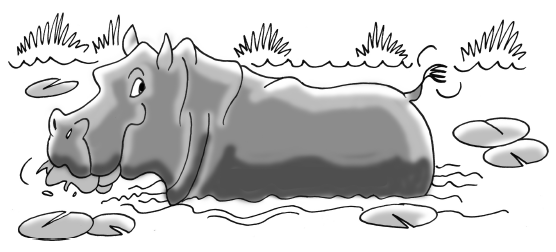### Home > AC > Chapter 5 > Lesson 5.1.4 > Problem5-42

5-42.Harry the Hungry Hippo is munching on the lily pads in his pond. When he got to the pond, there were $20$ lily pads, but he is eating $4$ lily pads an hour. Heinrick the Hungrier Hippo found a better pond with $29$ lily pads! He eats $7$ lily pads every hour.

1. If Harry and Heinrick start eating at the same time, when will their ponds have the same number of lily pads remaining?

Write an equation representing each hippo.

Set the two equations equal to each other and solve for $x$.

$x=3\ \text{hours}$

2. How many lily pads will be left in each hole at that time?

Substitute $3$ back into one of the original equations and solve for $y$.

Complete the tables in the eTool below to graph the results.
Click the link at right for the full version of the eTool: AC 5-42 HW eTool